# DeepFool — A simple and accurate method to fool deep Neural Networks.

Source: Deep Learning on Medium

## An Adversarial Attack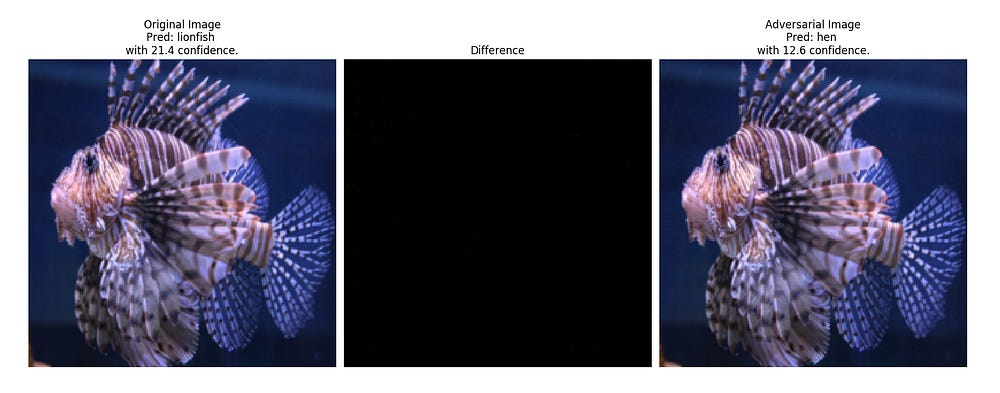Fig 1. Its not a fish, its a bird :) [Confidences shown are the values of logits and not passed through softmax]

Summary of the paper
DeepFool: A Simple and Accurate Method to Fool Deep Neural Networks
by
Seyed-Mohsen Moosavi-Dezfooli, Alhussein Fawzi, Pascal Frossard
Link to the paper:
https://arxiv.org/pdf/1511.04599.pdf

### Overview

Deep Neural Networks achieve state of the art performances in many tasks but fail miserably on slightly perturbed images, perturbed in a meaningful way (and not randomly).

The DeepFool paper have the following major contributions:

1. Simple and accurate method for computing the robustness of different classifiers to adversarial perturbations.
2. Experiments showing
– DeepFool computes a more optimal adversarial perturbation
– Adversarial Training significantly increases the robustness.

### DeepFool for binary classifiers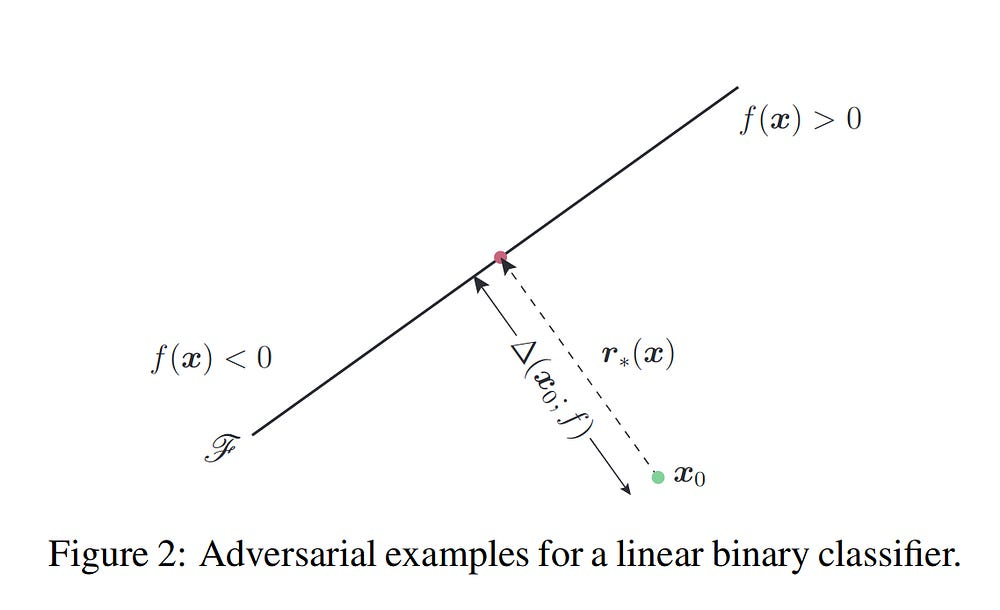FIg 2. Simple Image with Linear model to explain what DeepFool does.

It can be easily seen using a linear binary classifier, that the robustness of the model (f) for an input x_{0}is equal to the distance of x_{0} to the hyperparameter plane (which seperates the 2 classes).

Minimal perturbation to change the classifier’s decision corresponds to the orthogonal projection of x_{0} onto the hyperparameter plane. Given by: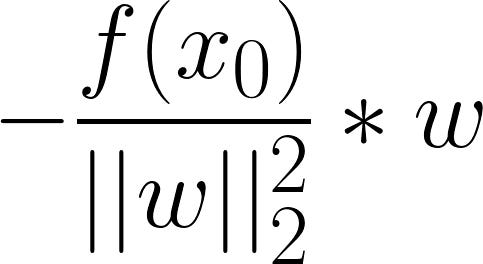Fig 3. Equation to calculate the minimal perturbation.

Below is the algorithm for DeepFool for binary classifiers: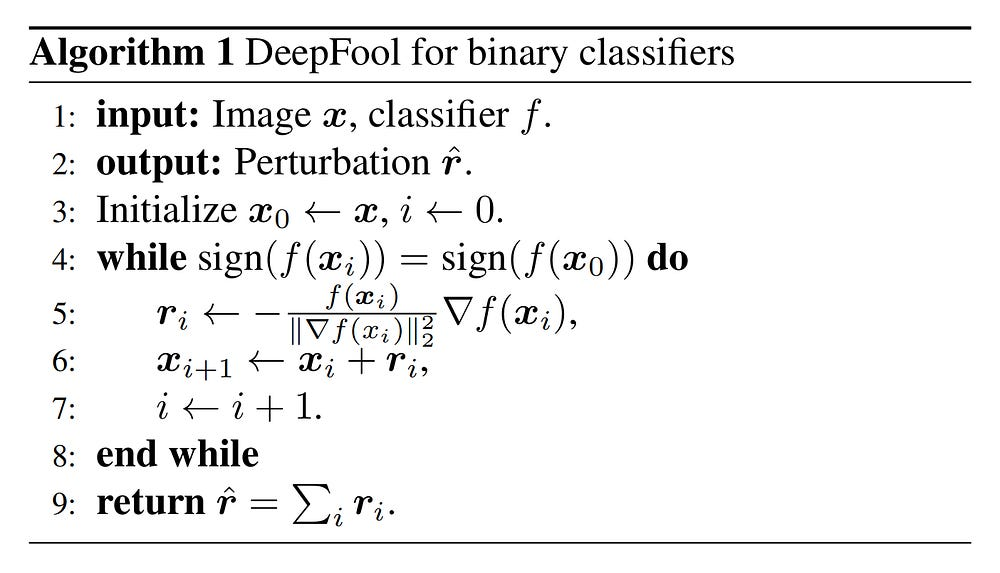Fig 4. Algorithm to calculate the Adversarial Image for Binary Classifiers.

Let’s go over the Algorithm:
1. The algorithm takes an input x and a classifier f .
2. Outputs the minimal perturbation required to miclassify the image.
3. Initialize the adversarial image with the original input. And the loop variable to 1.
4. Start and continue loop while the true label and the label of the adversarially perturbed image is the same.
5. Calculate the projection of the input onto the closest hyperplane. (minimal perturbation)
6. Add that perturbation to the image and test.
7–8. Increment Loop Variable; End Loop
9. Return the minimal perturbation

### DeepFool for Multiclass Classifiers

With multiclass classifiers, let’s say the input is x and for each class there is a hyperplane (straight plane that divides one class from the others) and based on the place in the space where x lies it is classified into a class. Now, all this algorithm does is, it finds the closest hyperplane, and then projects x onto that hyperplane and pushes it a bit beyond, thus misclassifying it with the minimal perturbation possible. That’s it. (Go through the section 3 in the paper to get a in-depth understanding)

Let’s take a look at the algorithm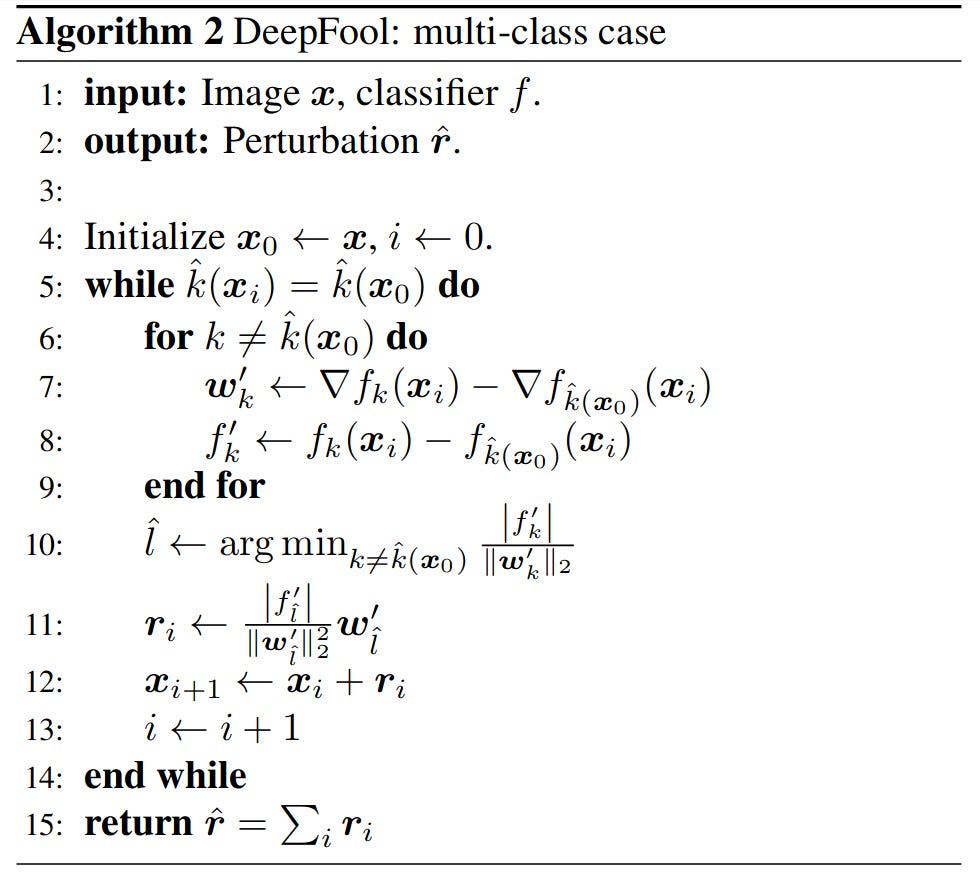Fig 5. Algorithm to calculate the Adversarial Image for Multiclass Classifiers.

Let’s quickly walkthrough each step of the algorithm:
1. Input is an image x and the classifier f which is the model.
2. The output which is the perturbation
3. [Blank]
4. We initialize the perturbed image with the original image and the loop variable.
5. We start the iteration and continue for uptil the original label and the perturbed label are not equal.
6–9. We consider n classes that had a the most probability after the original class and we store the minimum difference between the original gradients and the gradients of each of these class (w_{k}) and the difference in the labels (f_{k}).
10. The inner loop stores the minimum w_{k} and f_{k}, and using this we calculate the closest hyperplane for the input x(See Fig 6. for the formula of calculating the closest hyperplane)
11. We calculate the minimal vector that projects x onto the closest hyperplane that we calculated in 10.
12. We add the minimal perturbation to the image and check if its miclassified.
13–14. Loop variable increased; End Loop
15. Return the total perturbation, which is a sum over all the calculated perturbations.

Below is the equation to calculate the closest hyperplane: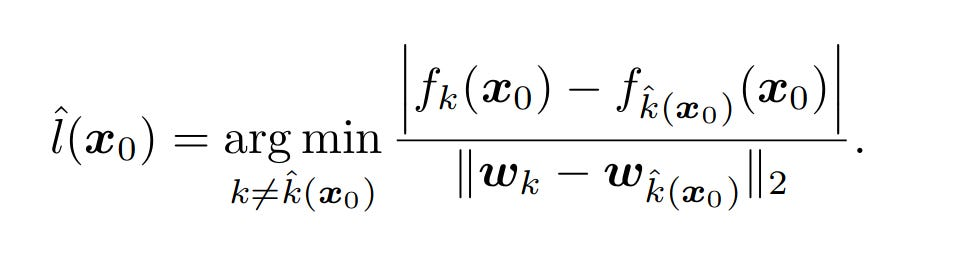Fig 6. Equation to calculate the closest hyperplane.

where,
variables starting with f are the class labels
variables starting with w are the gradients
where, the varibles with k as subscript are for the classes with the most probability after the true class and the variables with subscript \hat{k}(x+{0}) is for the true class.

Below is the equation to calculate the minimal perturbation (the vector which projects the input on the closest hyperplane)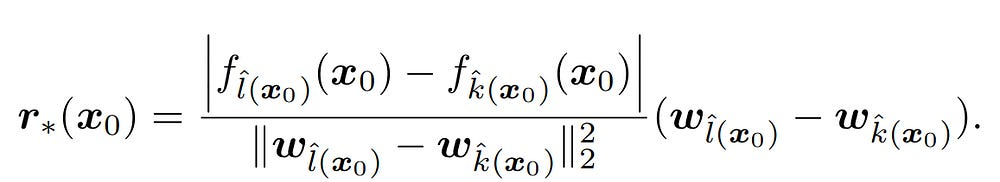Fig 7. Equation to calculate the minimal perturbation for multiclass classifiers.

#### Adversarial Robustness of a model

The DeepFool algorithm also provides a way to measure the adversarial robustness of an algorithm. It is proided by

# For images with a batch size of 1num_images = len(tloader))adversarial_robustness = (1 / num_images) * ((torch.norm(rt.flatten()) / (torch.norm(x.flatten()))

### Experiments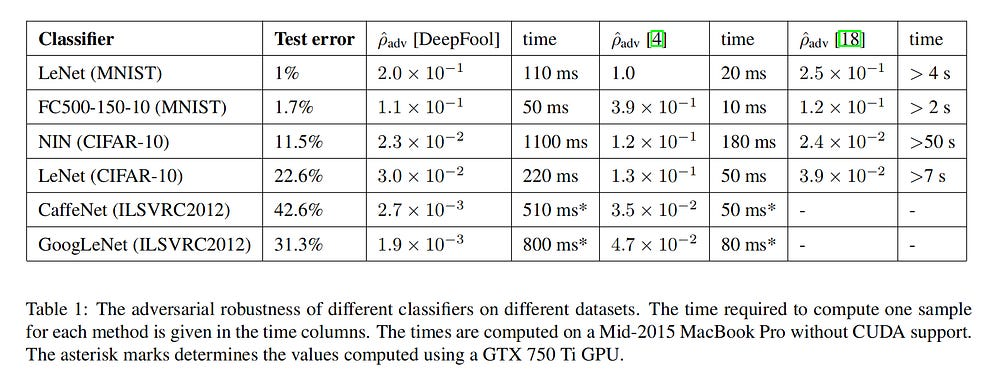Fig 8. Table demonstrating the test error rates and robustness of the models using each attack and the time to generate one adversarial example with each attack.

where,
4 — is the fast gradient sign method.
18 — is the attack from the paper titled Intriguing properties of neural networks by Szegedy et al.

Graphs showing the effect of adversarial training using adversarial images generated with the Fast Gradient Sign Method and DeepFool. These graphs demonstrates the importance of training with adversarial examples that are minimally perturbed (and not overly perturbed as in case of the Fast Gradient Sign Method.)

Graph which proves that training with overly perturbed images reduces the robustness of the model. In this experiment, the authors use DeepFool only with a gradually increasing value of alpha (where alpha is the value which is multiplied with the produced perturbation)

Table showing the test error rate after fine-tuning the network with different methods. This table shows that tuning the network with overly perturbed images leads to higher test error rates (adversarial images produced by Fast Gradient Sign Method.)

Graph showing the importance of using an accurate metric for calculating the adversarial robustness of a model. In this experiment they calculate the robustness of the NIN(Network-in-Network) model using the p_adv of the FGM attack and the DeepFool attack. The graph shows how the robustness calculated using the FGM attack gives a wrong measure as it really isn’t that robust as the previous examples show (and also the blue line which is for the robustness calculated using the DeepFool attack). Also, do note, how on the first extra epoch (the networks are trained for 5 extra epochs with adversarially perturbed images after original training with normal imges) the red-line (FGM) is not sensitive enough to demonstrate the loss of robustness.

### References

1. Seyed-Mohsen Moosavi-Dezfooli, Alhussein Fawzi, Pascal Frossard; The IEEE Conference on Computer Vision and Pattern Recognition (CVPR), 2016, pp. 2574–2582

Hope the article was clear enough to give a good understanding of what the DeepFool Algorithm is and how it works. I wish to update this article in future if I find more intuitive explanations or areas that needs more focus. Do please read the paper to get a better understanding. Thanks for reading!